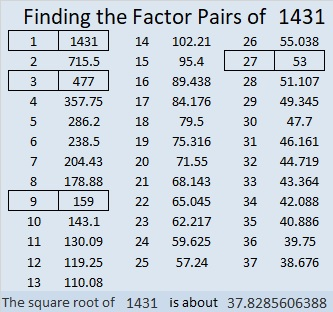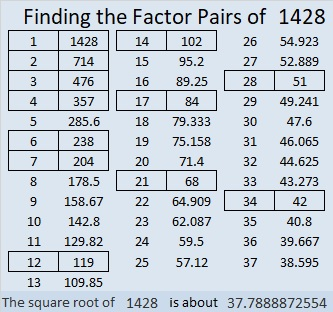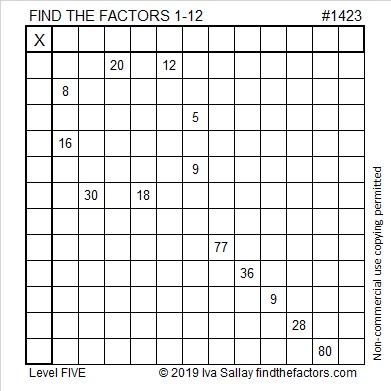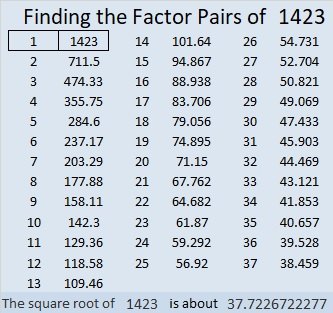# 1431 is a Triangular Number and a Hexagonal Number

If you only look at a list of triangular numbers or a list of hexagonal numbers, you might miss the relationship that figurate numbers have with each other.

1378 is the 52nd triangular number, and you can use it to find the 53rd triangular number (1431), the 53rd square number, the 53rd pentagonal number, and so forth.

351 is the 26th triangular number, and you can use it to find the 27th triangular number, the 27th square number, the 27th pentagonal number, the 27th hexagonal number (1431), and so forth.

See the relationship in the graphic below:Should you get excited that 1431 is BOTH a triangular number and a hexagonal number? Not really. It turns out that every hexagonal number is also a triangular number. (But not every triangular number is a hexagonal number.)

Here are a few more facts about the number 1431:

• 1431 is a composite number.
• Prime factorization: 1431 = 3 × 3 × 3 × 53, which can be written 1431 = 3³ × 53
• 1431 has at least one exponent greater than 1 in its prime factorization so √1431 can be simplified. Taking the factor pair from the factor pair table below with the largest square number factor, we get √1431 = (√9)(√159) = 3√159
• The exponents in the prime factorization are 3 and 1. Adding one to each exponent and multiplying we get (3 + 1)(1 + 1) = 4 × 2 = 8. Therefore 1431 has exactly 8 factors.
• The factors of 1431 are outlined with their factor pair partners in the graphic below.1431 is the hypotenuse of a Pythagorean triple:
756-1215-1431 which is 27 times (28-45-53)

# 1428 Factor Trees in Autumn

I recently decided that I wanted to make some factor trees in various fall colors. 1428 has plenty of factors so it has MANY different factor trees. Here are just eleven of them, each initially factored by a different factor pair.Here are some more facts about the number 1428:

• 1428 is a composite number.
• Prime factorization: 1428 = 2 × 2 × 3 × 7 × 17, which can be written 1428 = 2² × 3 × 7 × 17
• 1428 has at least one exponent greater than 1 in its prime factorization so √1428 can be simplified. Taking the factor pair from the factor pair table below with the largest square number factor, we get √1428 = (√4)(√357) = 2√357
• The exponents in the prime factorization are 2, 1, 1, and 1. Adding one to each exponent and multiplying we get (2 + 1)(1 + 1)(1 + 1)(1 + 1) = 3 × 2 × 2 × 2 = 24. Therefore 1428 has exactly 24 factors.
• The factors of 1428 are outlined with their factor pair partners in the graphic below.1428 is the hypotenuse of a Pythagorean triple:
672-1260-1428 which is (8-15-17) times 84.

# 1423 Lollipop

Lollipops are candies that easily delight children. Will today’s lollipop-wannabe puzzle be a delight?Print the puzzles or type the solution in this excel file: 12 Factors 1419-1429

The puzzle number is 1423. Here are a few facts about that number:

• 1423 is a prime number.
• Prime factorization: 1423 is prime.
• 1423 has no exponents greater than 1 in its prime factorization, so √1423 cannot be simplified.
• The exponent in the prime factorization is 1. Adding one to that exponent we get (1 + 1) = 2. Therefore 1423 has exactly 2 factors.
• The factors of 1423 are outlined with their factor pair partners in the graphic below.How do we know that 1423 is a prime number? If 1423 were not a prime number, then it would be divisible by at least one prime number less than or equal to √1423. Since 1423 cannot be divided evenly by 2, 3, 5, 7, 11, 13, 17, 19, 23, 29 31, or 37, we know that 1423 is a prime number.